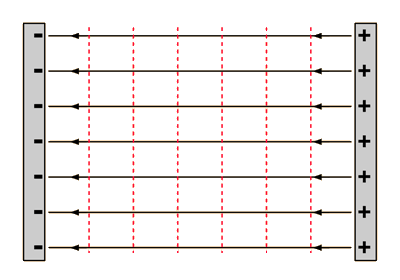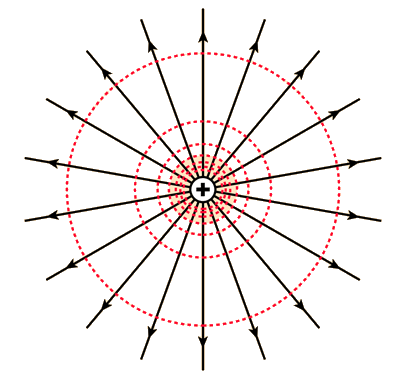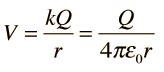# Equipotential Lines

Equipotential lines are like contour lines on a map which trace lines of equal altitude. In this case the "altitude" is electric potential or voltage. Equipotential lines are always perpendicular to the electric field. In three dimensions, the lines form equipotential surfaces. Movement along an equipotential surface requires no work because such movement is always perpendicular to the electric field.Index

Voltage concepts

 HyperPhysics***** Electricity and Magnetism R Nave
Go Back

# Equipotential Lines: Constant FieldFor parallel conducting plates like those in a capacitor, the electric field lines are perpendicular to the plates and the equipotential lines are parallel to the plates.
 Other charge geometries
Index

Voltage concepts

 HyperPhysics***** Electricity and Magnetism R Nave
Go Back# Equipotential lines: point charge

The electric potential of a point charge is given byso that the radius r determines the potential. The equipotential lines are therefore circles and a sphere centered on the charge is an equipotential surface. The dashed lines illustrate the scaling of voltage at equal increments - the equipotential lines get further apart with increasing r.

 Other charge geometries
Index

Voltage concepts

 HyperPhysics***** Electricity and Magnetism R Nave
Go Back# Equipotential lines: dipole

The electric potential of a dipole show mirror symmetry about the center point of the dipole. They are everywhere perpendicular to the electric field lines. The plane perpendicular to the line between the charges at the midpoint is an equipotential plane with potential zero.

 Other charge geometries
Index

Voltage concepts

Electric dipole concepts

 HyperPhysics***** Electricity and Magnetism R Nave
Go Back